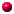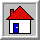﻿ Congjun Wu's homepage Congjun Wu's homepage

Unconventional magnetism and dynamic generation of spin-orbit coupling

Spin-orbit coupling is conventionally viewed as a single-electron property inherited from relativity but not directly related to many-body interactions. We with (S. C. Zhang) proposed a new concept for spontaneous generation of spin-orbit coupling purely from electron interactions ( [Ref. 1] ). It is through an unconventional type of magnetic phase transitions (e.g. e-wave) based on Fermi surface instabilities. ( [Ref. 2] ).

##Uncoventional magnetism of itinerant electrons

In ferromagnetic metals, spin rotational symmetry is broken because of the spontaneous spin polarization. However, orbital rotational symmetry remains, i.e., spin of each momentum polarizes along the same direction around Fermi surfaces. This is similar to the situation in conventional s-wave superconductors where the phase of the pairing gap functions keeps constant over the Fermi surface. Therefore ferromagnetism can be considered as s-wave" magnetism. In the context of superconductivity or fermion pairing superfluidity, in addition to the conventional s-wave pairing, there are unconventional pairing structures, including the d-wave pairing in high Tc cuprates and the p-wave pairing in superfluid-He3 and Sr2RuO4.

In analogy to unconventional superconductivity, we have proposed non-$s$-wave'' generalizations of ferromagnetic states in which spin no longer polarizes along a unique direction but varies with momentum ( [Ref. 2] ). These unconventional magnetic states have close connections to many research focuses in condensed matter physics, including unconventional superconductivity, spin-orbit coupling and spintronics, and electron liquid crystal states in strongly correlated systems. The unconventional magnetic states include both isotropic and anisotropic cases. The isotropic phases still have circular or spherical Fermi surfaces with topologically non-trivial spin configurations in momentum space, providing a mechanism for dynamic generation of spin-orbit coupling through many-body interactions; the anisotropic phases are electron liquid crystal states with spin degree of freedom, exhibiting anisotropic Fermi surface distortions. Both types of phases arise from a general class of Fermi liquid instabilities of the Pomeranchuk type in the spin channel, which include ferromagnetism as a specital example.

##Pomeranchuk instability of Fermi liquids

Most of our current understanding of interacting electron systems are based on the Landau theory of Fermi lqiuids. In this theory, the interactions among quasiparticles are captured by a few Landau parameters $F^{s,a}_l$, where $l$ denotes the orbital angular momentum partial-wave channel, and $s$, $a$ denote spin-singlet and -triplet channels, respectively. Physical quantities, such as the spin susceptibility, and properties of collective excitations, such as the dispersion relation of zero sound collective modes, acquire significant but finite renormalizations due to the Landau interactions. It has, however, long been known that the stability of Fermi liquids requires that the Landau parameters cannot be too negative, $F^{s,a}_l>-(2l+1)$, a result derived by Pomeranchuk. Otherwise, Fermi surfaces will be distorted. Such a class of Fermi surface instabilities are named Pomeranchuk instabilities. The most familiar examples of these Pomeranchuk instabilities are found in the s-wave channel: the Stoner ferromagnetism at $F^a_1<-1$ channel and phase separation at $F^s_1<-1$.

##Dynamic spin-orbit ordering: Alpha and beta-phases

The unconventional magetism arises from the Pomeranchuk instabilities in the spin triplet channel with high orbital partical waves $(F^a_l (l>0))$. The resultant ordered phases are classified into two classes, dubbed the alpha and beta phases by analogy to the superfluid He3-A and B-phases, respectively.

The Fermi surfaces in the $\alpha$-phases exhibit spontaneous anisotropic distortions, which are the electronic nematic phases augmented by the spin degree of freedom as shown in Fig. \ref{fig:phase} ($C$). This phase in the $p$-wave channel was proposed by J. Hirsch (PRB 41, 6820; PRB 41, 6828) under the name of "spin-split" state in the Chromium system. The Fermi surfaces in the beta-phases remain circular or spherical with topologically non-trivial spin configurations in momentum space. The beta-phases are isotropic, but exhibit relative spin-orbit symmetry breaking, a concept first proposed by Leggett in the superfluid He-3 systems. The mean-field band structures in both phases exhibit various types of spin-orbit couplings. These states have close connections to many research directions in condensed matter physics, including unconventional superconductivity, spin-orbit coupling and spintronics, and electron liquid crystal states in strongly correlated systems. In particular, the recent experiment by D. Hsieh's group found a parity-breaking electronic nematic state in Cd$_2$Re$_2$O$_7$, which may be related to this class of phase.

##Spin-orbit coupled Fermi liquid theory

We have investigated Fermi liquid states of the ultracold magnetic dipolar Fermi gases in the simplest two-component case [Ref. 3]. The magnetic dipolar interaction is invariant under the simultaneous spin-orbit rotation but not under either the spin or the orbit one. Therefore, the corresponding Fermi liquid theory is intrinsically spin-orbit coupled. This is a fundamental feature of magnetic dipolar Fermi gases different from electric dipolar ones. The Landau interaction matrix is calculated and is diagonalized in terms of the spin-orbit coupled partial-wave channels of the total angular momentum J. An exotic propagating collective mode is identified as spin-orbit coupled Fermi surface oscillations in which spin distribution on the Fermi surface exhibits a topologically nontrivial hedgehog configuration.

References and talks

• 1. Congjun Wu, and Shou-cheng Zhang, "Dynamic generation of spin-orbit coupling", Phys. Rev. Lett. 93, 36403(2004) , see pdf file .

• 2. Congjun Wu, Kai Sun, Eduardo Fradkin, and Shou-Cheng Zhang, "Fermi liquid instabilities in the spin channel" , Phys. Rev. B 75, 115103 (2007), see pdf file.

• 3. Yi Li, Congjun Wu, "Spin-orbit coupled Fermi liquid theory with magnetic dipolar interaction",
Phys. Rev. B 85, 205126 (2012)
, pdf file

• Talk: Unconventional magnetism and dynamic spin-orbit orderingBack to home